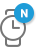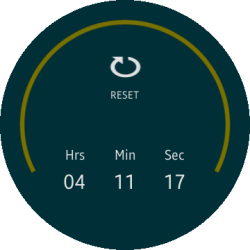# Rotary Timer Sample OverviewThe (Circle) Rotary Timer sample application demonstrates how you can implement a circular digital clock with a timer function in your application.

The following figure illustrates the main views of the (Circle) Rotary Timer sample application.

Figure: (Circle) Rotary Timer main viewsClick the hour, minute, and second values to set up the timer. To start the timer, click START.

The timer is stops when the number in the digital clock is reduced to 0. To set another time, click RESET.

## Implementation

To implement the circular rotary timer:

To use the rotary function, the application must include the efl_extension.h header file. To detect rotary events, add the necessary callback functions.

```eext_rotary_object_event_activated_set(ad->layout, EINA_TRUE);
2. Create the layout for the timer preview.

Use the _layout_create() function to create the main layout of the application, and add a digital clock to display the timer preview.

```static Evas_Object *
{
_D("Layout create");
Evas_Object *layout = NULL;

retv_if(!layout, NULL);

// ad->timer_edj_path == app_get_resource_path() + /edje/timer.edj
evas_object_size_hint_weight_set(layout, EVAS_HINT_EXPAND, EVAS_HINT_EXPAND);

evas_object_show(layout);

return layout;
}```
3. Initialize the application and set the timer.

Use the digital_create() function to create the digital clock and set the quantity of time for the timer.

```void
{
_D("Digital create");
Evas_Object *left_col = NULL;
Evas_Object *right_col = NULL;

ret_if(!left_col);
evas_object_size_hint_weight_set(left_col, EVAS_HINT_EXPAND, EVAS_HINT_EXPAND);
evas_object_size_hint_align_set(left_col, EVAS_HINT_FILL, EVAS_HINT_FILL);

ret_if(!right_col);
evas_object_size_hint_weight_set(right_col, EVAS_HINT_EXPAND, EVAS_HINT_EXPAND);
evas_object_size_hint_align_set(right_col, EVAS_HINT_FILL, EVAS_HINT_FILL);
}```
4. Start the timer.

The _start_clicked_cb() callback starts the timer. When you click START, the application calculates the quantity of time using the _calc_setting_time() function and displays the total time. The _timer_start_cb() function is called every second to reduce the number in the digital clock.

```static void
_start_clicked_cb(void *data, Evas_Object *obj, void *event_info)
{
{

return;
}

// Make the digital clock unclickable

// Reduce 1 second every second
}

static Eina_Bool
_timer_start_cb(void *data)
{
// Get the previous time
post_second_time = atoi(pre_second_time);
post_minute_time = atoi(pre_minute_time);
post_hour_time = atoi(pre_hour_time);
// Reduce 1 second
if (post_second_time == 0)
{
if (post_minute_time == 0)
{
post_second_time = 59;
post_minute_time = 59;
post_hour_time = post_hour_time - 1;
}
else
{
post_second_time = 59;
post_minute_time = post_minute_time - 1;
}
}
else
{
post_second_time = post_second_time - 1;
}
// Display the new time

free(hour_val);
free(minute_val);
free(second_val);

return ECORE_CALLBACK_RENEW;
}```
5. Reset the timer.

To set another time when the user clicks RESET, call the _reset_clicked_cb() callback function.

```static void
{